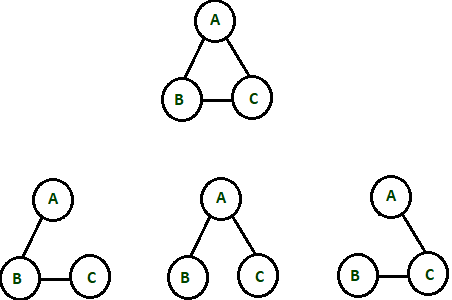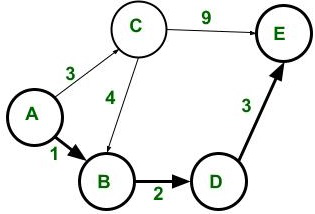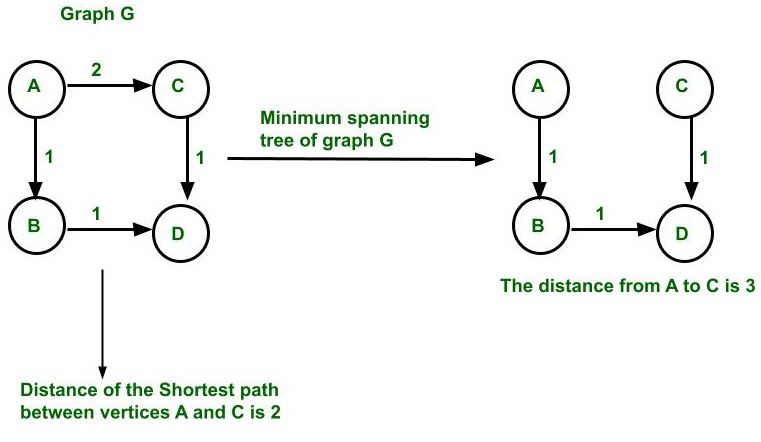# Difference between Minimum Spanning Tree and Shortest Path

• Last Updated : 01 Jul, 2021

Spanning tree: A spanning tree (T) of an undirected graph(G) is a subgraph which is a tree that includes all the vertices of a graph (G) and the minimum number of edges required to connect the graph (G). And it is a known maximal set of edges with no cycles.

Properties:

• If a graph(G) is not connected then it does not contain a spanning tree (i.e. it has many spanning-tree forests).
• If a graph(G) has V vertices then the spanning tree of that graph G has V-1 edges.
• In the case of a complete (Kn) undirected, labeled, and unweighted graph, the number of possible spanning trees can be found out By using Cayley’s formula: Kn = nn-2.
• For the K3 graph (i.e. complete graph of 3 vertices) the number of possible spanning trees is 33-2 = 31= 3.Possible spanning trees in K3 graph

• The number of minimum spanning trees of a graph other than a complete graph can find out by using the Kirchhoff theorem.

The Shortest path:Shortest path(A, B, D, E) between vertices A and E in the weighted directed graph

• The shortest path between any two vertices (say between A and E) in a graph such that the sum of weights of edges that are present in the path (i.e. ABDE) is minimum among all possible paths between A and E.
• The shortest path can find out for graphs which are directed, undirected or mixed.
• The problem for finding the shortest path can be categorized as
• Single-source the shortest path: In this, the shortest path is calculated from a source vertex to all other vertices present inside the graph.
• Single-destination the shortest path: In this, the shortest path is calculated from all vertices in the directed graph to a single destination vertex. This can be converted into a single pair with the shortest path problem by reversing the edges of the directed graph.
• All pairs the shortest path: In this, the shortest path is calculated between every pair of vertices.

The followings are the difference between the Minimum spanning tree(MST) and the Shortest path:My Personal Notes arrow_drop_up